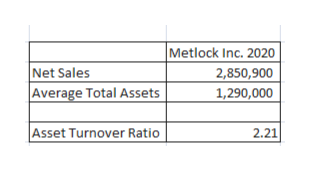# During 2020, Metlock, Inc. reported net sales of \$2,850,900 and net income of \$1,550,000. Its balance sheet reported average total assets of \$1,290,000.Calculate the asset turnover. (Round answer to 2 decimal places, e.g. 1.25.)

Question

During 2020, Metlock, Inc. reported net sales of \$2,850,900 and net income of \$1,550,000. Its balance sheet reported average total assets of \$1,290,000.

Calculate the asset turnover. (Round answer to 2 decimal places, e.g. 1.25.)

check_circle

Step 1

Asset turnover ratio calculates how much the company was able to generate revenue using it's assets. Hence, the formula of Asset turnover ratio = Net Sales/Average Assets, where average assets = Beginning Assets+ Ending Assets/2

Step 2

We can calculate the asset turnover ratio by dividing Net Sales by average total ass...help_outlineImage TranscriptioncloseMetlock Inc. 2020 Net Sales Average Total Assets 2,850,900 1,290,000 Asset Turnover Ratio 2.21 fullscreen

### Want to see the full answer?

See Solution

#### Want to see this answer and more?

Solutions are written by subject experts who are available 24/7. Questions are typically answered within 1 hour.*

See Solution
*Response times may vary by subject and question.
Tagged in